# Indexes in Oracle with Examples

## Indexes in Oracle with Examples

In this article, I am going to discuss Indexes in Oracle with Examples. Please read our previous article where we discussed the basic concepts of Synonyms in Oracle. Until now we have learned about synonyms and sequences. Now, we will try to learn about indexes.

##### What are Indexes in Oracle?

Indexes are the schema objects that act like a pointer to the database. A pointer to the table. It is a private area in the memory to speed up the performance of queries. This can reduce the disk input/output (I/O) by using a rapid path access method to locate data quickly. This is dependent on the table. This is used and maintained automatically by the Oracle server. No direct activity is required by the user.

An index provides direct and fast access to rows in a table. Its purpose is to reduce the dis I/O by using an indexed path to locate the data quickly. Indexes are logically and physically independent of the data in the objects with which they are associated. This means that they can be created or dropped at any time, and have no effect on the base tables or other indexes.

##### We have two ways to create an index:
• The first way is when we create a primary key in a table, then Oracle automatically creates the unique index. The unique index indicates that these values should be unique.
• The other way is to create the index manually. We can create unique or non-unique indexes on columns to speed up access to the rows.
##### You can create two types of indexes.
• Unique index: The Oracle server automatically creates this index, when you define a column in a table to have a PRIMARY KEY or a UNIQUE constraint. The name of the index is the name that is given to the constraint.
• Non-Unique index: This is an index that a user can create. For example, you can create the FOREIGN KEY column index for a join-in query to improve the speed of retrieval.

For example, we are querying a table by always using a search condition. In that case, we can create an index on a particular column to run the query faster.

Note: When we drop a table, then indexes corresponding to the table are also dropped.

Syntax: CREATE {UNIQUE} [BITMAP] INDEX Index_Name on table (column{, column}..);

Example: create index emp_last_name_idx on employees (last_name);

Now, let’s try to learn about automatic index creation. Let’s try to create a table with the primary key.

```CREATE TABLE EMP_IND
( EMPNO NUMBER CONSTRAINT EMP_IND_PK PRIMARY KEY,
ENAME VARCHAR2(100) UNIQUE,
NICKNAME VARCHAR2(100),
email varchar2(100)
);
```

We can see the table name is EMP_IND and we have columns EMPNO, ENAME, NICKNAME, and email. So, the column EMPNO is the primary key constraint. So, whenever there is a primary key then the index will automatically be created. We also have ENAME which is unique. Here we haven’t mentioned the constraint and name. So, this will automatically be SYS_C. Oracle will automatically create indexes for unique key constraints. Let’s try to create the table EMP_IND.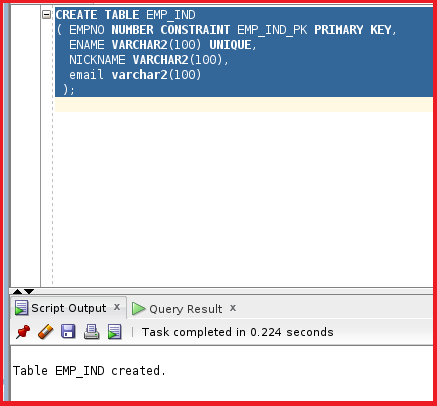So, the table EMP_IND is created. Let’s try to insert two values in the table EMP_IND.

```INSERT INTO EMP_IND (EMPNO,ENAME,NICKNAME,EMAIL) VALUES ('1','Ahmed Samer','Ahmed.Samer','Ahmed.Samer@gmail.com');
INSERT INTO EMP_IND (EMPNO,ENAME,NICKNAME,EMAIL) VALUES ('3','Khaled Ali','Khaled.Ali','Khaled.Ali@hotmail.com');
INSERT INTO EMP_IND (EMPNO,ENAME,NICKNAME,EMAIL) VALUES ('4','Hassan Nabil','Hassan.Nabil','Hassan.Nabil@yahoo.com');
COMMIT;
```

We are trying to insert four values here with empno, ename, nickname, and email. Let’s try to insert these records.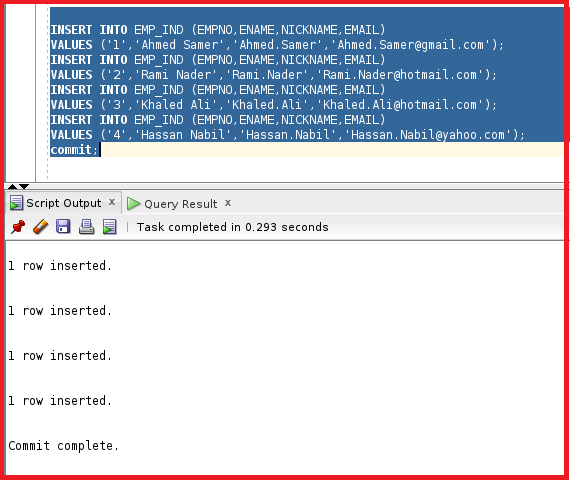So, the records are inserted into the table. The oracle will implicitly create indexes for the Primary key, and Unique key, and the the index will be the same as the name of the constraint name. We can check the details of the indexes using the dictionary USER_INDEXES.

SELECT * FROM USER_INDEXES WHERE TABLE_NAME=’EMP_IND’;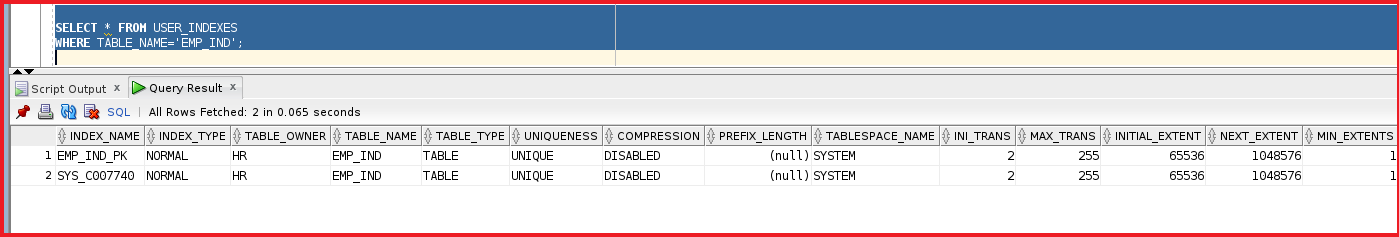We can see there are two indexes EMP_IND_PK, SYS_C007740. These are the unique indexes associated with the table EMP_IND. Here we cannot find the column name. We cannot find the column names in this dictionary. We can find that using the dictionary USER_IND_COLUMNS.

select * from user_ind_columns where table_name=’EMP_IND’;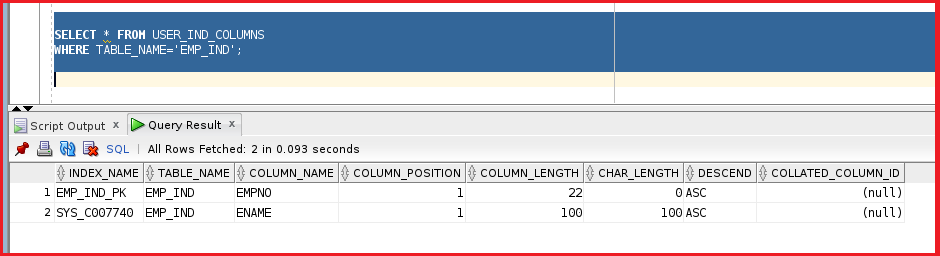We can see the column name in the dictionary user_ind_columns. From the above image, we can see the column_name for the indexes. We can see other details like column_position and column_length. We can see the column_position is 1.

When we try to query the table EMP_IND, the query will be faster, we cannot identify that is faster because it is a small table. When we try to execute a larger table that has millions of rows then we can feel the difference. Let’s try to test that by running a query on the EMP_IND table.

select * from EMP_IND where EMPNO=1;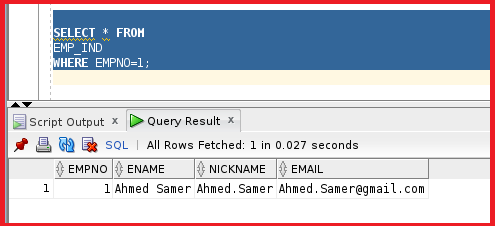So, the query is obtained. We can check the Explain Plan to see if the query is picking the indexes or not. We can check the Explain Plan by clicking the boxed icon in the below image.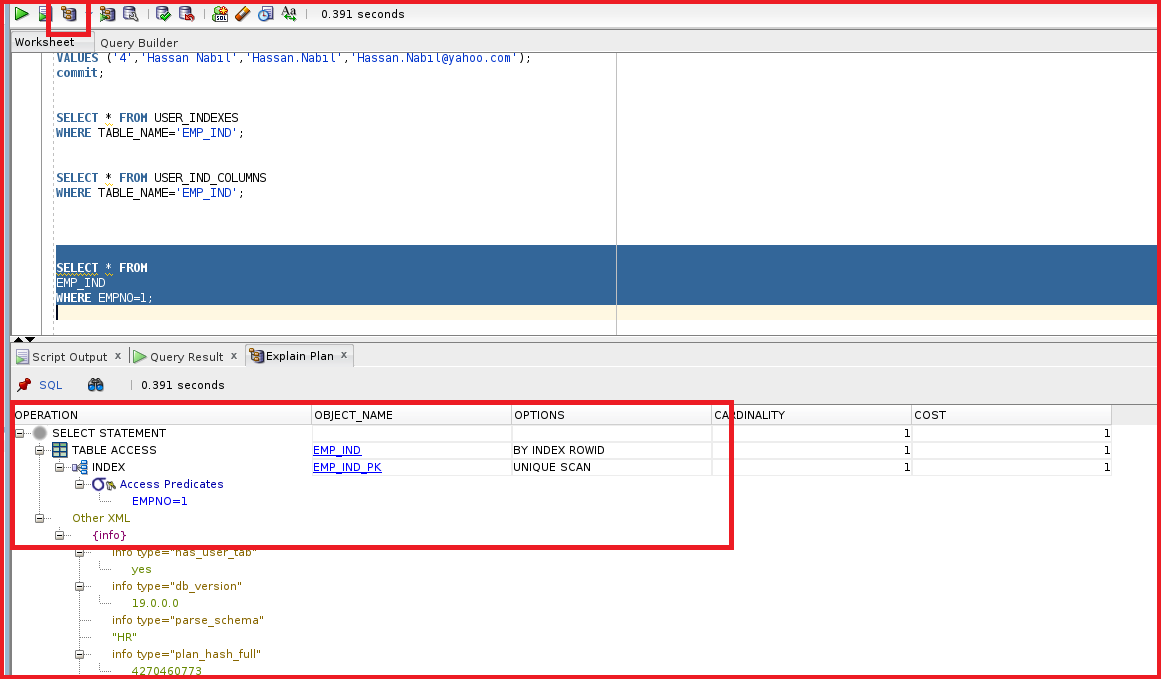We can see the Explain Plan for the query “ select * from EMP_IND where EMPNO=1”. There is an index mentioned in the select statement using the index EMP_IND_PK. So, we can understand that Oracle is using the index to query the output in the table EMP_IND. Let’s try to use another example and see the uses of an index.

SELECT * FROM EMP_IND WHERE ename=’Ahmed Samer’;

Let’s try to execute this query and see the result.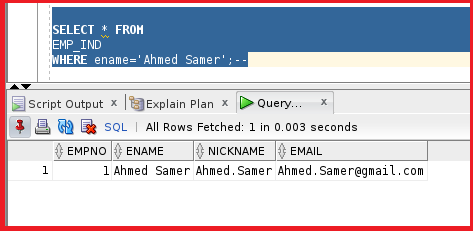We got the result of the query. Now, let’s try to look at the Explain Plan for this query.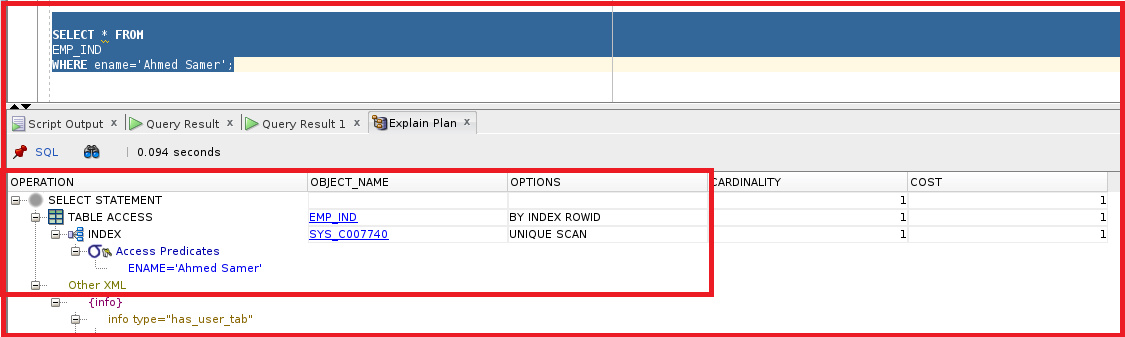We can see the query is using the index SYS_C007740. This query is also using the index because we have mentioned the column name ENAME. We have created the constraints for both EMPID and ENAME. So, we do not have an index on the nickname column. Let’s try to run a query where the index is not present.

SELECT * FROM EMP_IND WHERE NICKNAME=’Ahmed.Samer’;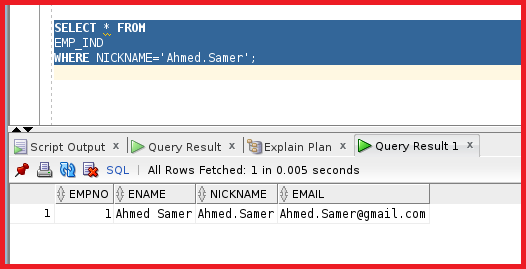We have used the column nickname because we haven’t created any index on the column NICKNAME. Let’s try to create an index on this column NICKNAME.

CREATE INDEX EMP_IND_NICKNAME ON EMP_IND(NICKNAME);

In order to create an index, we need to have the ‘CREATE INDEX’ privilege. The DBA will be granting this privilege. Let’s go ahead and create the index.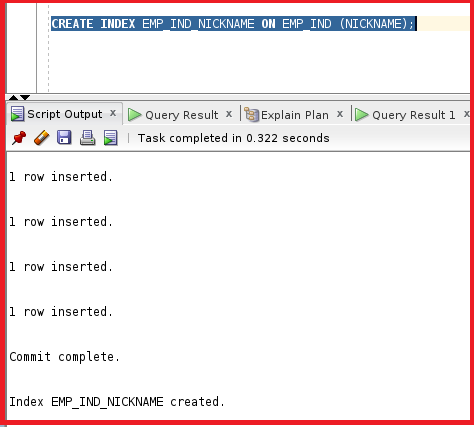So, the index is now created. Let’s now try to check the index details using the dictionary USER_INDEXES.

SELECT * FROM USER_INDEXES where TABLE_NAME=’EMP_IND’;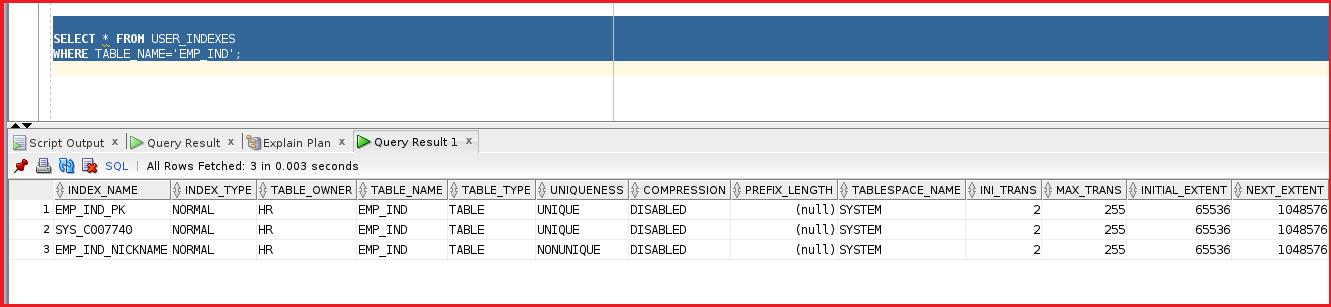So, we can see the new index name EMP_IND_NICKNAME. We can see the uniqueness is mentioned as NONUNIQUE. This is normal because we can two people under the same nickname. Let’s try to test this index by querying the table EMP_IND using the NICKNAME column.

SELECT * FROM EMP_IND WHERE NICKNAME=’Ahmed.Samer’;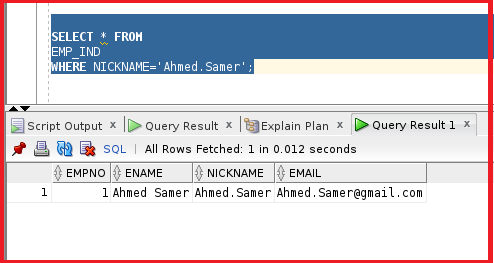So, the query is working fine.

##### What is the difference between an index and a unique index in Oracle?

When a unique index is on the table, this means we are creating a unique constraint on the table which means the column should be doubled. Let’s try to test that with the column EMAIL. Let’s try to create a unique index on the column email because we cannot find two people using the same email address.

CREATE UNIQUE INDEX EMP_IND_EMAIL ON EMP_IND (EMAIL);

We can create a unique index without creating a unique constraint. We have created the unique index with the name EMP_IND_EMAIL in the column EMAIL. Let’s try to create the index.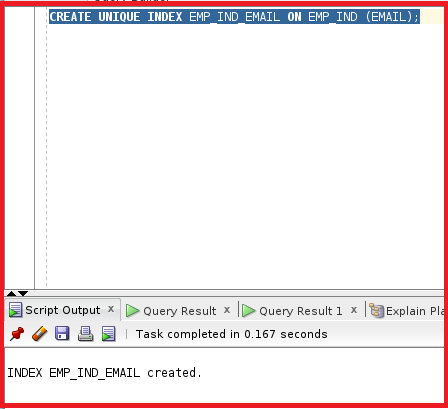So, the index is created. This will directly act as a unique constraint. Let’s now try to add a new row with the same email. Let’s now try to test that index by creating a new user with the same email.

`INSERT INTO EMP_IND (EMPNO, ENAME, NICKNAME, EMAIL) VALUES ('10','karem Samer','Ahmed.Samer','Ahmed.Samer@gmail.com');`

So, we are using the same email with a different ename. Let’s try to check this insert statement.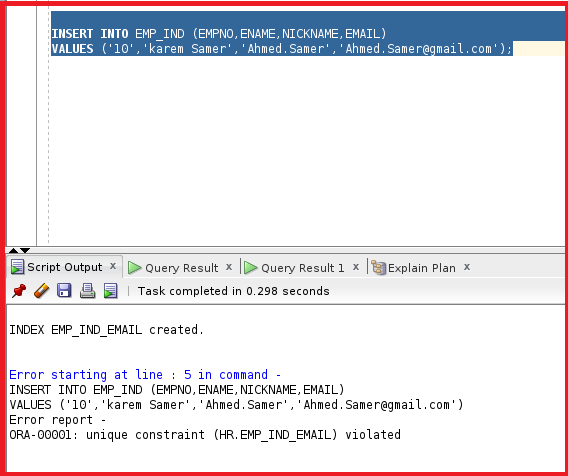We can see the error unique constraint violated. This will be working the same as the constraint. We can also create another index for the ENAME column but using the function-based index upper (ENAME). The index creation is not only for the column, we can also create if for the expression.

```SELECT * FROM
EMP_IND
WHERE upper(ename)='AHMED SAMER';```

We have mentioned the upper(ename) in the query. In order to use that upper function, we can use that in the index so that whenever we use the function upper then the index will be used. Let’s try to create an index for this function.

CREATE INDEX EMP_IND_UP_ENAME ON EMP_IND (UPPER(ENAME));

We have mentioned the upper function for the column ENAME. Let’s try to create the index.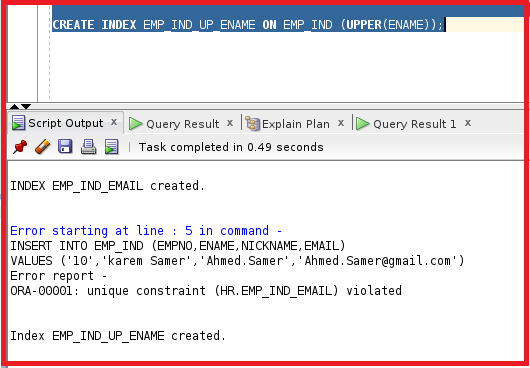So, the index is created. Now, let’s try to check the index details with the data dictionary USER_INDEXES.

select * from USER_INDEXES where TABLE_NAME=’EMP_IND’;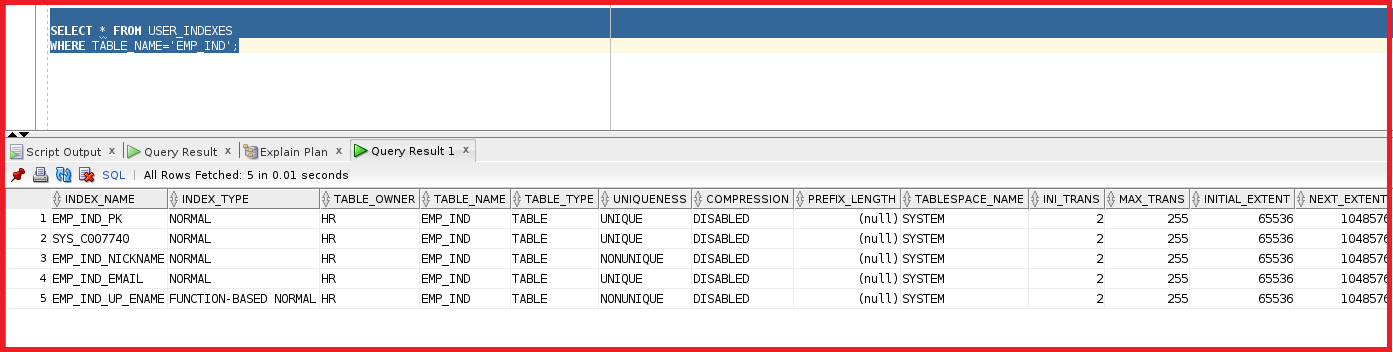We can see the index details here. The index name is EMP_IND_UP_ENAME. The index type is mentioned as FUNCTION BASED NORMAL because we are creating the index on the function, not on the column name. Now, if we try to check the USER_IND_COLUMNS the column name will not be displayed.

SELECT * FROM USER_IND_COLUMNS WHERE TABLE_NAME=’EMP_IND’;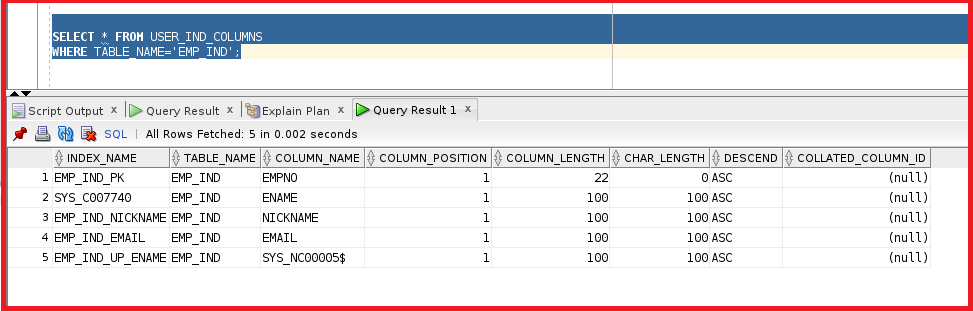We can see the EMP_IND_UP_ENAME as the index name but if we check the column name it shows SYS_NC. This indicates this is not a column this is an expression. In order to get the details of this expression we must check the other data dictionary. USER_IND_EXPRESSIONS.

SELECT * FROM USER_IND_EXPRESSIONS WHERE TABLE_NAME=’EMP_IND’;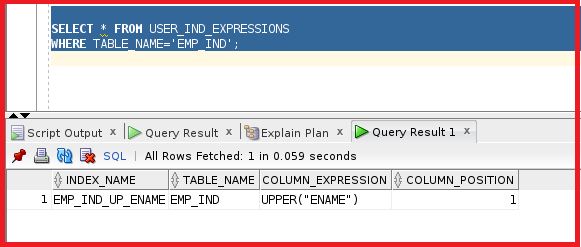Here we can check the column expression. We can see the index name. So, we can understand and get information about the index using three indexes.

• USER_INDEXES
• USER_IND_COLUMNS
• USER_IND_EXPRESSIONS

So, let’s try to check the index by running the query.

```SELECT * FROM
EMP_IND
WHERE UPPER(ENAME)='AHMED SAMER';```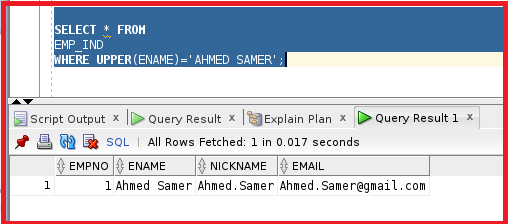We can see the output. So, this also working fine. We have learned about the indexes and index creation and data dictionary to check the indexes.

##### Index Creation Guidelines in Oracle

We can create an index but there are few rules to create an index. Below is a list of rules that are required while creating indexes.

##### Create an index when:
• A column containing a wide range of values
• A column contains a large number of null values
• One or more columns are frequently used together in a WHERE clause or a join condition.
• The table is large and most queries are expected to retrieve less than 2% to 4% of the rows in the table.
##### Do not create an index when:
• The columns are not often used as a condition in the query.
• The table is small or most queries are expected to retrieve more than 2% to 4% of the rows in the table.
• The table is updated frequently.
• The indexed columns are referenced as part of an expression.

Let’s try to do some practices about index creation.

##### Naming the index while creating the table:

When we create a primary key or unique key while creating the table, then Oracle will automatically create an index with the same name. Without automatically creating the index by Oracle we can create an index manually while creating the table.

```CREATE TABLE EMP_IND1
( EMPNO NUMBER CONSTRAINT EMP_IND1_PK PRIMARY KEY USING INDEX
(create index EMP_IND1_ind on EMP_IND1 (EMPNO)  ),
FNAME VARCHAR2(100),
lname VARCHAR2(100),
EMAIL VARCHAR2(100),
gender char(1)
);
```

We have a column EMPNO as the primary key. We don’t want the unique indexes with the name EMP_IND1_PK. We need another name for this primary key. So, we can create the index as below.

```EMPNO NUMBER CONSTRAINT EMP_IND1_PK PRIMARY KEY USING INDEX
(create index EMP_IND1_ind on EMP_IND1 (EMPNO).
```

So, we can create this way while creating the table. So, after the primary key, we need to mention “USING INDEX” and we have to create the index. We do have other columns like FNAME, lname, email, and gender. Let’s try to create the EMP_IND1.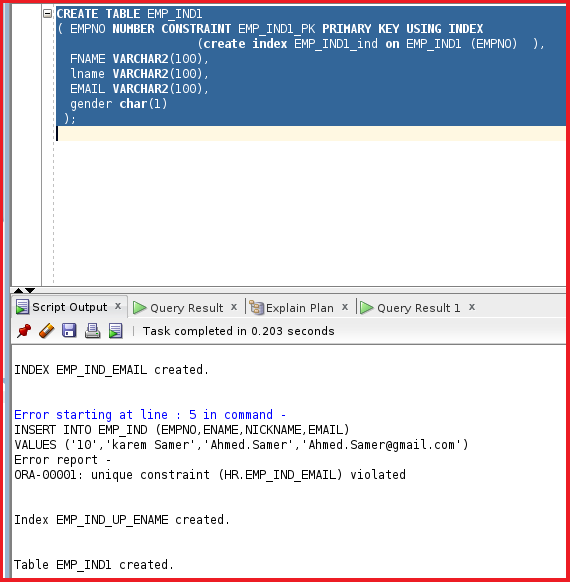So, the table EMP_IND1 is created. We can create an index of composite columns.

CREATE INDEX EMP_IND1_COMP ON EMP_IND1 (FNAME,LNAME);

Let’s try to create the index EMP_IND1_COMP.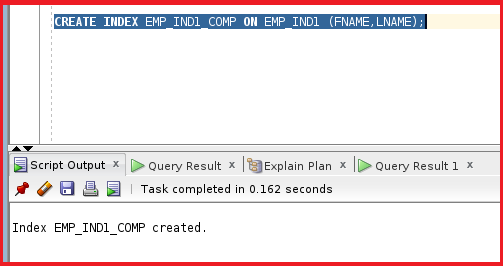So, the index is created. Let’s now try to check the index details.

SELECT * FROM USER_INDEXES WHERE TABLE_NAME=’EMP_IND1′;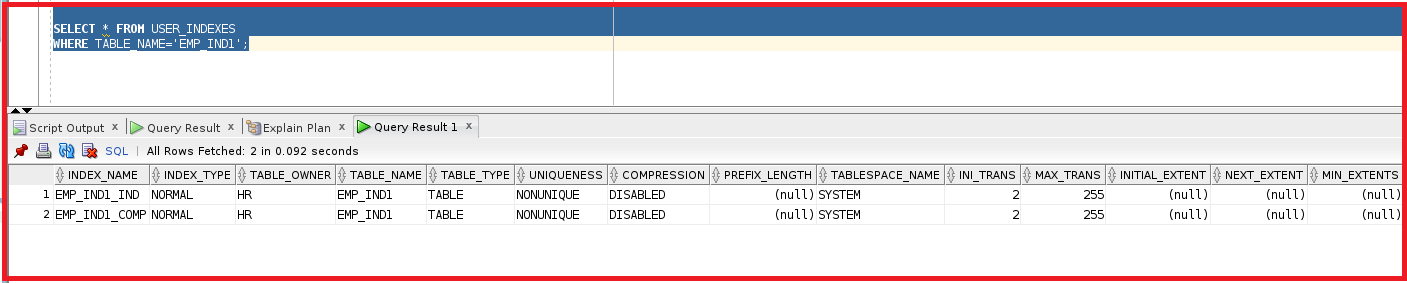We can see the index details here with index names EMP_IND1_IND, EMP_IND1_COMP. These indexes are non-unique indexes. The indexes are different from the name of primary constraints. Let’s have a look at the column names associated with the indexes.

SELECT * FROM USER_IND_COLUMNS WHERE TABLE_NAME=’EMP_IND1′;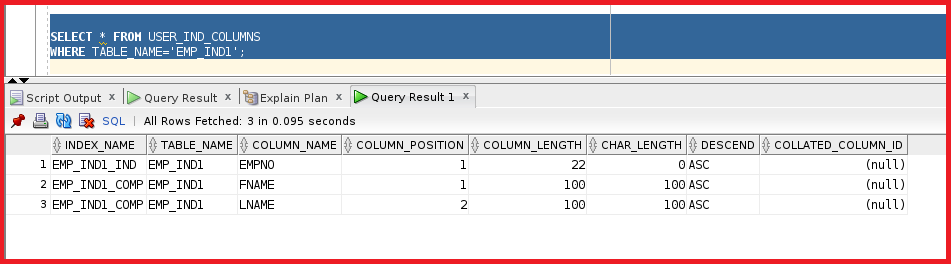We can see the indexes EMP_IND1_IND. We have an index containing composite columns fname and lname which is a composite index.

##### BITMAP Index in Oracle:

To specify a BITPAM index indicate that the index is to be created with a bitmap for each distinct key, rather than indexing each row separately. Bitmap indexes store rowids associated with a key value as a bitmap.

In some cases when we create an index, we are creating a pointer to a row, but in some cases, if there is a table where the column has gender it differentiates between MALE and FEMALE. It is not efficient to make unique or non-unique indexes pointing to each row. It is better to create a bitmap index for the group of columns at a single index.

Syntax: CREATE BITMAP INDEX EMP_IND_b ON EMP_IND1 (GENDER);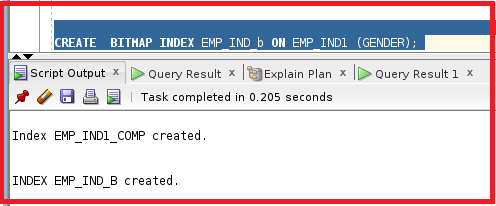So, the bitmap index is created. This means the pointer will be on a distinct value not on each row. We can drop the index by using the below statement.

DROP INDEX EMP_IND1_COMP;

So, we have learned good knowledge about the indexes. We mostly use indexes to increase the performance of the queries.

In the next article, I am going to discuss Exception Handling in Oracle with Examples. Here, in this article, I try to explain Indexes in Oracle with Examples. I hope you enjoy this Indexes in Oracle article.